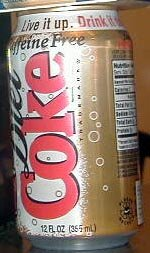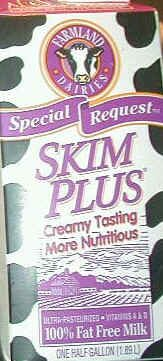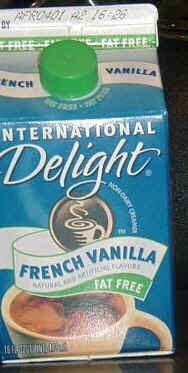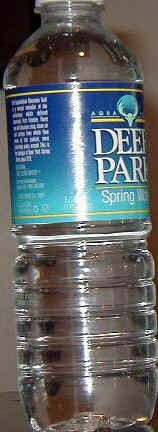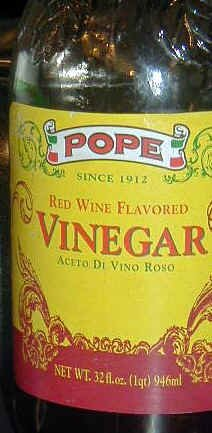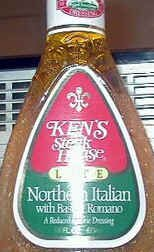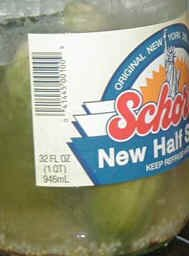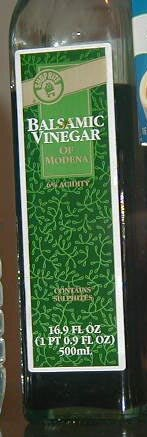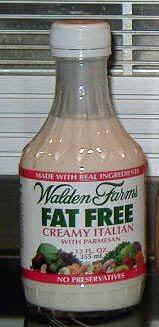# LITERS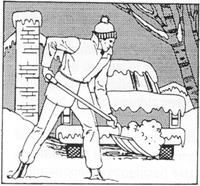25 Degrees Fahrenheit

COMMON PREFIXES
(to be used with basic units)
milli: one-thousandth (0.001)
centi:one-hundredth (0.01)
kilo: one-thousand times (1000)

For example:
1000 millimeters = 1 meter
100 centimeters = 1 meter
1000 meters = 1 kilometer

##### 1 LITER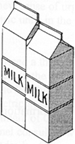##### 1 QUART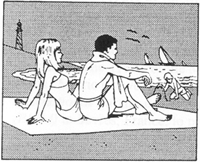25 Degrees Celsius
OTHER COMMONLY USED UNITS
millimeter: 0.001 meter - diameter of a paper clip wire
centimeter: 0.01 meter - a little more than the width of a paper clip (about 0.4 inch)
kilometer: 1000 meters - somewhat further than 1/2 mile (about 0.6 miles)
kilogram: 1000 grams - a little more than 2 pounds (about 2.2 pounds)
milliliter: 0.001 liter - five of them make a teaspoon

OTHER USEFUL UNITS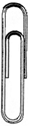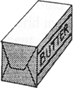1 pound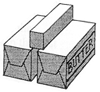1 Kilogram

A liter is a measurement of volume.  It tells you how much liquid is inside a container.

1 liter = 1000 cubic centimeters
(That is a box that is 10 cm long, 10 cm wide, and 10 cm high.)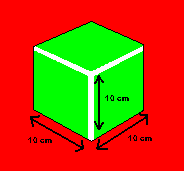or

1 liter = 1 cubic decimeter
(That is a box that is 1 dm long, 1 dm wide, and 1 dm high.)

Here two most common metric measures used for liquids are:

LITER          and        MILLILITER

A milliliter is about how much liquid an eyedropper can hold.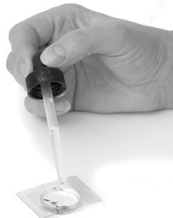= 1 milliliter

The best way to understand how much liquid a liter will hold
is to make a liter box yourself.

1.  Create a template like this on construction paper in which each square is 10 cm x 10 cm).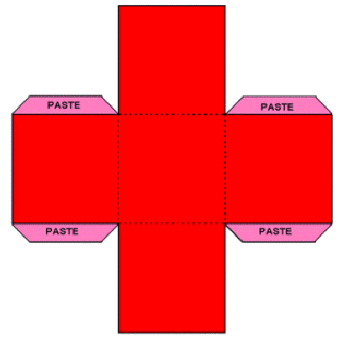2.  Cut on the solid lines.
3.  Fold on the dotted lines and fold back the paste tabs.
4.  Use glue and tape to hold the box together.
5.  Put a plastic bag inside the box to use as a liner.

Your completed liter box should look something like this: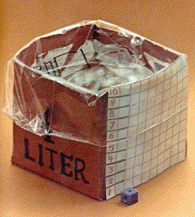6.  Experiment with different amounts of liquid to see how much 1 liter will hold.

Here are some containers I found in my kitchen.
Can you determine what the metric label on each one says?